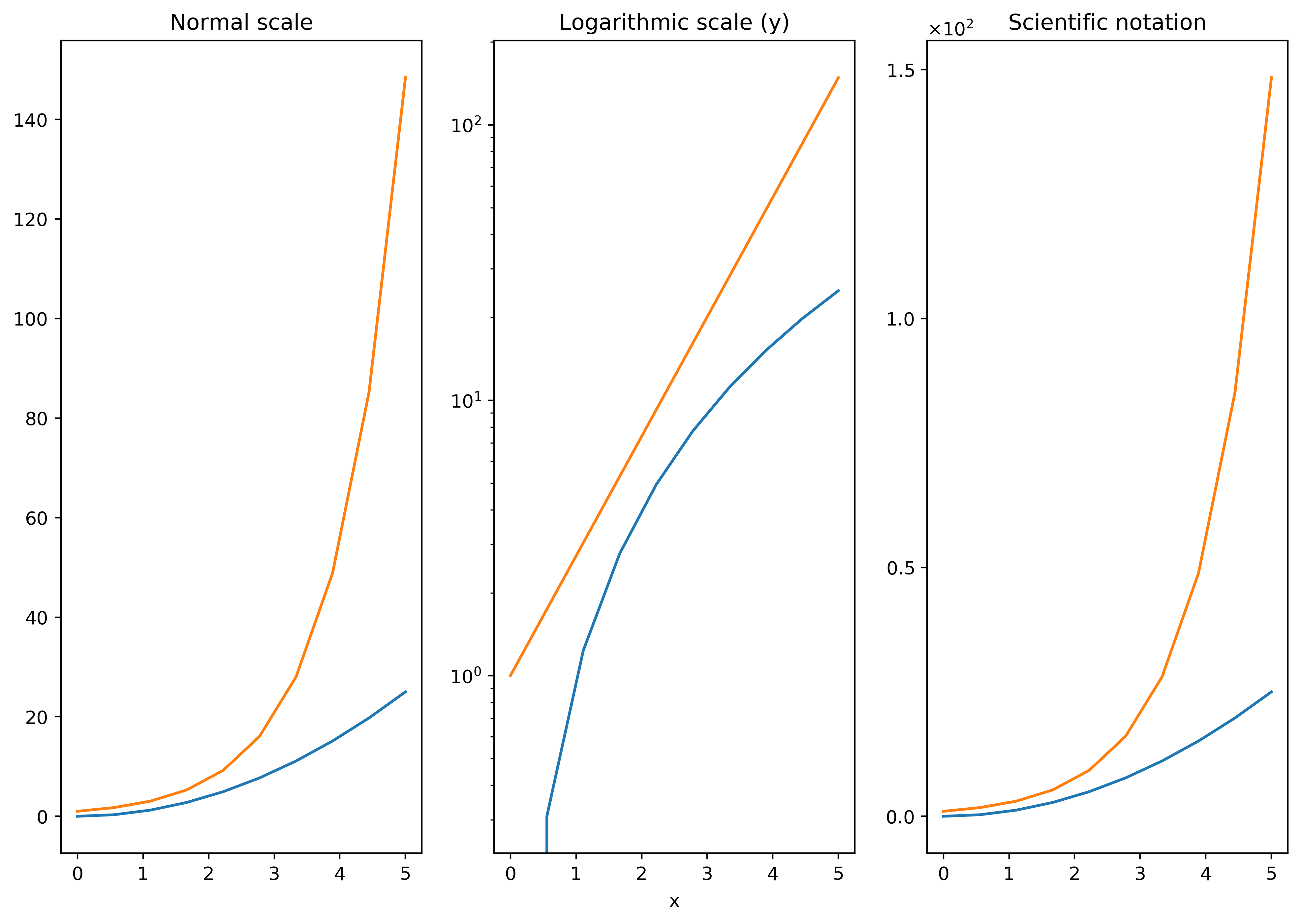# 对数刻度

16 篇文章 1 订阅
import matplotlib.pyplot as plt
import numpy as np
from pylab import *
fig,axes=plt.subplots(1,3,figsize=(12,8))
x=linspace(0,5,10)

axes.plot(x,x**2,x,exp(x))
axes.set_title('Normal scale')

axes.plot(x,x**2,x,exp(x))
axes.set_yscale('log')
axes.set_xlabel('x')
axes.set_title('Logarithmic scale (y)')

axes.plot(x,x**2,x,exp(x))
axes.set_yticks([0,50,100,150])
axes.set_title('Scientific notation')
from matplotlib import ticker
#刻度容器
#y轴用科学计数法表示
formatter = ticker.ScalarFormatter(useMathText=True)
formatter.set_scientific(True)
formatter.set_powerlimits((-1,1))
axes.yaxis.set_major_formatter(formatter)

plt.savefig('E:\python\慕课网 python\.idea\photo\对数刻度.png',dpi=400,bbox_inches='tight')
plt.show()
print(matplotlib.rc_params())12-31321
09-0521万+
12-243267
12-06621
03-17102
09-26
04-19280
11-054861点击重新获取扫码支付余额充值